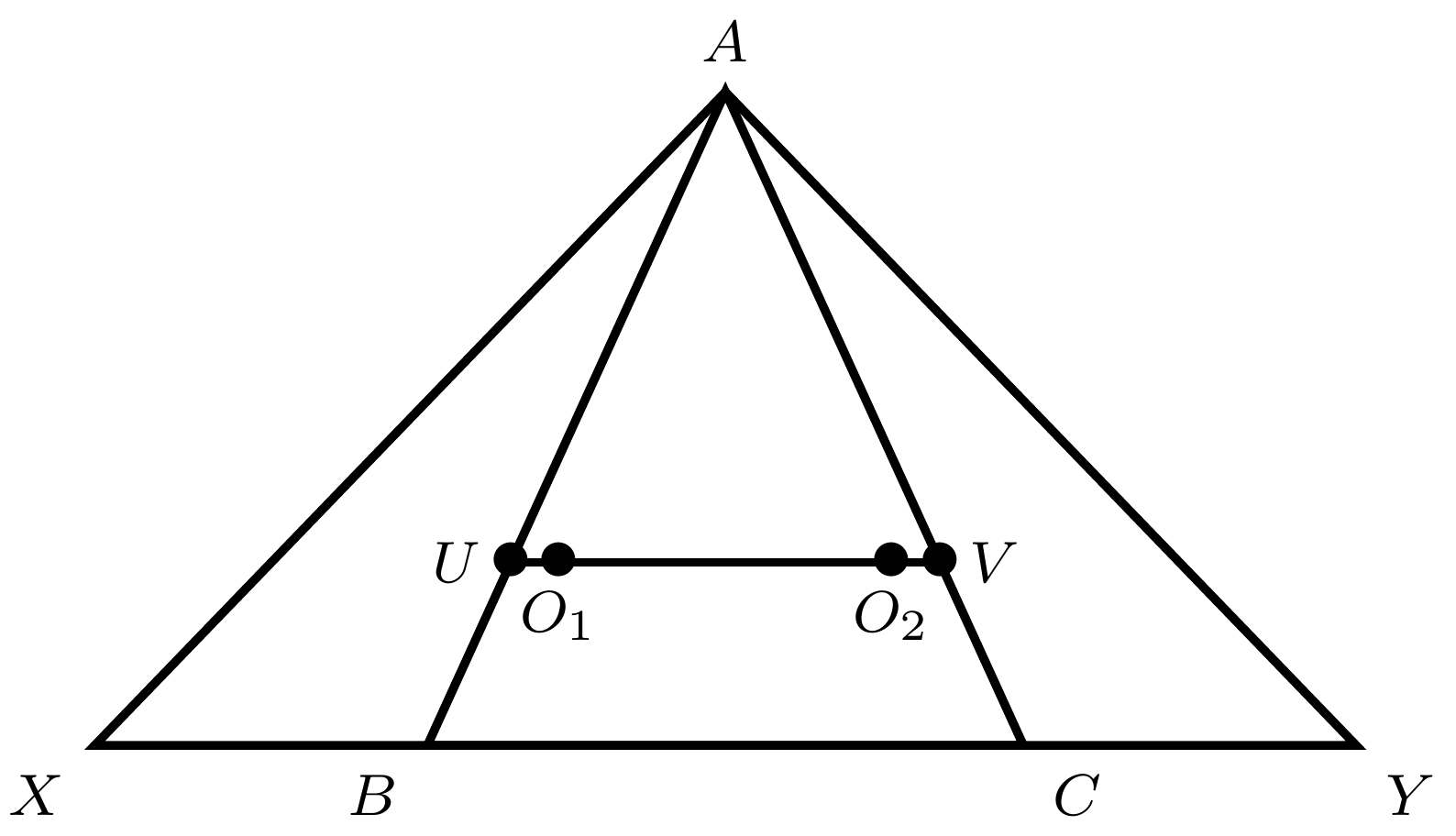###### back to index | new

Let $ABCDE$ be a pentagon inscribed in a circle such that $AB = CD = 3$, $BC = DE = 10$, and $AE= 14$. The sum of the lengths of all diagonals of $ABCDE$ is equal to $\frac{m}{n}$, where $m$ and $n$ are relatively prime positive integers. What is $m+n$ ?

A circle with center $C$ is tangent to the positive $x$ and $y$-axes and externally tangent to the circle centered at $(3,0)$ with radius $1$. What is the sum of all possible radii of the circle with center $C$?

A circle has a radius of $\log_{10}{(a^2)}$ and a circumference of $\log_{10}{(b^4)}$. What is $\log_{a}{b}$?

Angle $ABC$ of $\triangle ABC$ is a right angle. The sides of $\triangle ABC$ are the diameters of semicircles as shown. The area of the semicircle on $\overline{AB}$ equals $8\pi$, and the arc of the semicircle on $\overline{AC}$ has length $8.5\pi$. What is the radius of the semicircle on $\overline{BC}$?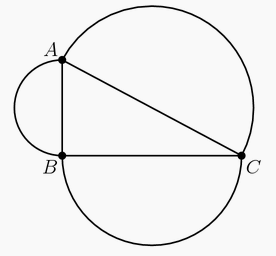Circle $A$ has radius $100$. Circle $B$ has an integer radius $r<100$ and remains internally tangent to circle $A$ as it rolls once around the circumference of circle $A$. The two circles have the same points of tangency at the beginning and end of cirle $B$'s trip. How many possible values can $r$ have?

Points $A$ and $C$ lie on a circle centered at $O$, each of $\overline{BA}$ and $\overline{BC}$ are tangent to the circle, and $\triangle ABC$ is equilateral. The circle intersects $\overline{BO}$ at $D$. What is $\frac{BD}{BO}$?

Point $H$ is the orthocenter of triangle $ABC$. Points $D, E$ and $F$ lie on the circumcircle of triangle $ABC$ such that $AD\parallel BE\parallel CF$. Points $S, T,$ and $U$ are the respective reflections of $D, E, F$ across the lines $BC, CA$ and $AB$. Prove that $S, T, U, H$ are cyclic.

Let $ABCD$ be a cyclic quadrilateral. Let $P, Q, R$ be the feet of the perpendiculars from $D$ to the lines $BC, CA$ and $AB$ respectively. Show that $PQ = QR$ iff the bisectors of $\angle ABC$ and $\angle ADC$ meet on $AC$.

In the diagram below, the circle with center $A$ is congruent to and tangent to the circle with center $B$. A third circle is tangent to the circle with center $A$ at point $C$ and passes through point $B$. Points $C, A$, and $B$ are collinear. The line segment $\overline{CDEFG}$ intersects the circles at the indicated points. Suppose that $DE=6$ and $FG=9$. Find $AG$.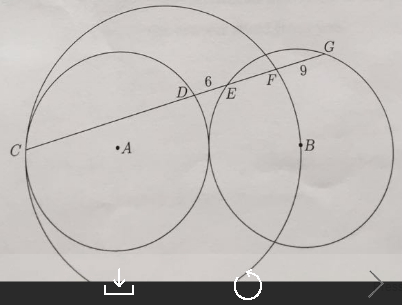A $45^\circ$ arc of circle A is equal in length to a $30^\circ$ arc of circle B. What is the ratio of circle A's area and circle B's area?

Let $AD$ be the altitude in $\triangle{ABC}$ from the vertex $A$. If $\angle{A}=45^\circ$, $BD=3$, $DC=2$, find the area of $\triangle{ABC}$.

Let $ABCD$ be a quadrilateral with an inscribed circle $\omega$ that has center $I$. If $IA=5$, $IB=7$, $IC=4$, $ID=9$, find the value of $\frac{AB}{CD}$.

There are $n$ circles inside a square $ABC$ whose side's length is $a$. If the area of any circle is no more than 1, and every line that is parallel to one side of $ABCD$ intersects at most one such circle, show that the sum of the area of all these $n$ circles is less than $a$.

A quadrilateral is inscribed in a circle of radius $200\sqrt{2}$. Three of the sides of this quadrilateral have length $200$. What is the length of the fourth side?

An isosceles triangle with equal sides of 5 and bases of 6 is inscribed in a circle. Find the radius of that circle.

As shown, two vertices of a square are on the circle and one side is tangent to the circle. If the side length of the square is 8, find the radius of the circle.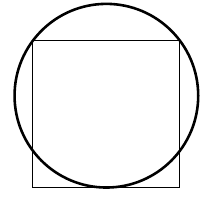$DEB$ is a chord of a circle such that $DE=3$ and $EB=5 .$ Let $O$ be the center of the circle. Join $OE$ and extend $OE$ to cut the circle at $C.$ Given $EC=1,$ find the radius of the circle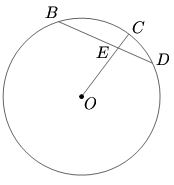Chords $AB$ and $CD$ of a given circle are perpendicular to each other and intersect at a right angle at point $E$. If $BE=16$, $DE=4$, and $AD=5$, find $CE$.

Let quadrilateral $ABCD$ inscribe a circle. If $BE=ED$, prove $$AB^2+BC^2 +CD^2 + DA^2 = 2AC^2$$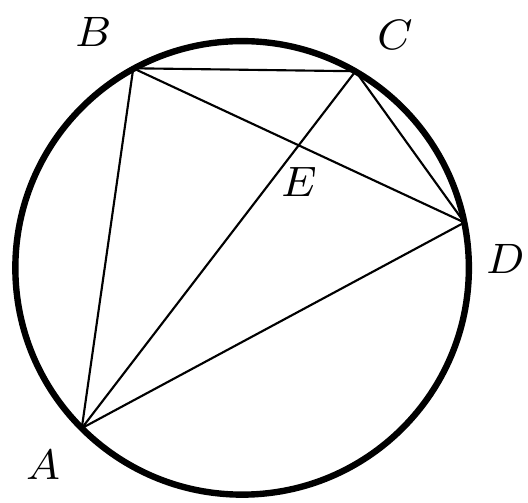As shown, $\angle{ACB} = 90^\circ$, $AD=DB$, $DE=DC$, $EM\perp AB$, and $EN\perp CD$. Prove $$MN\cdot AB = AC\cdot CB$$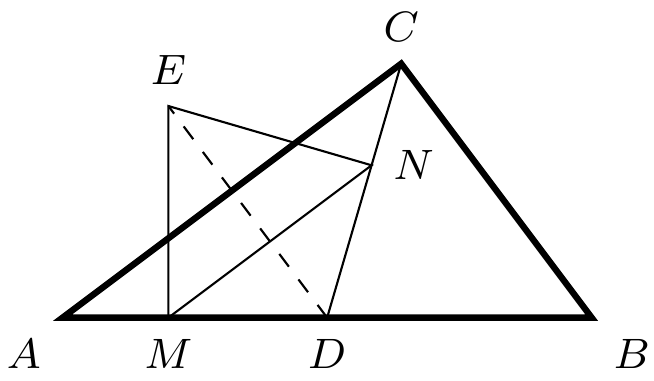Let $O_1$ and $O_2$ be two intersecting circles. Let a common tangent to these two circles touch $O_1$ at $A$ and $O_2$ at $B$. Show that the common chord of these two circles, when extended, bisects segment $AB$.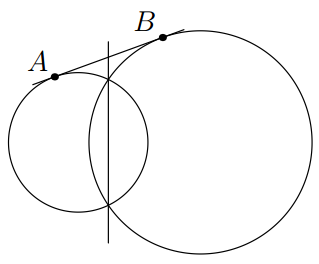Line $PT$ is tangent to circle $O$ at point $T$. $PA$ intersects circle $O$ and its diameter $CT$ at $B$, $D$, and $A$ in that order. If $CD=2, AD=3,$ and $BD=6$, find the length of $PB$.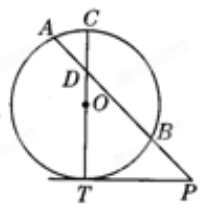Let $ABCD$ be a square. Point $E$ is outside $ABCD$ such that $DE=CD$ and $\angle{AED}=15^\circ$. Show $\triangle{DCE}$ is equilateral.

What is the Ptolemy Theorem?

As shown, points $X$ and $Y$ are on the extension of $BC$ in $\triangle{ABC}$ such that the order of these four points are $X$, $B$, $C$, and $Y$. Meanwhile, they satisfy the relation $BX\cdot AC = CY\cdot AB$. Let $O_1$ and $O_2$ be the circumcenters of $\triangle{ACX}$ and $\triangle{ABY}$, respectively. If $O_1O_2$ intersects $AB$ and $AC$ at $U$ and $V$, respectively, show that $\triangle{AUV}$ is isosceles.